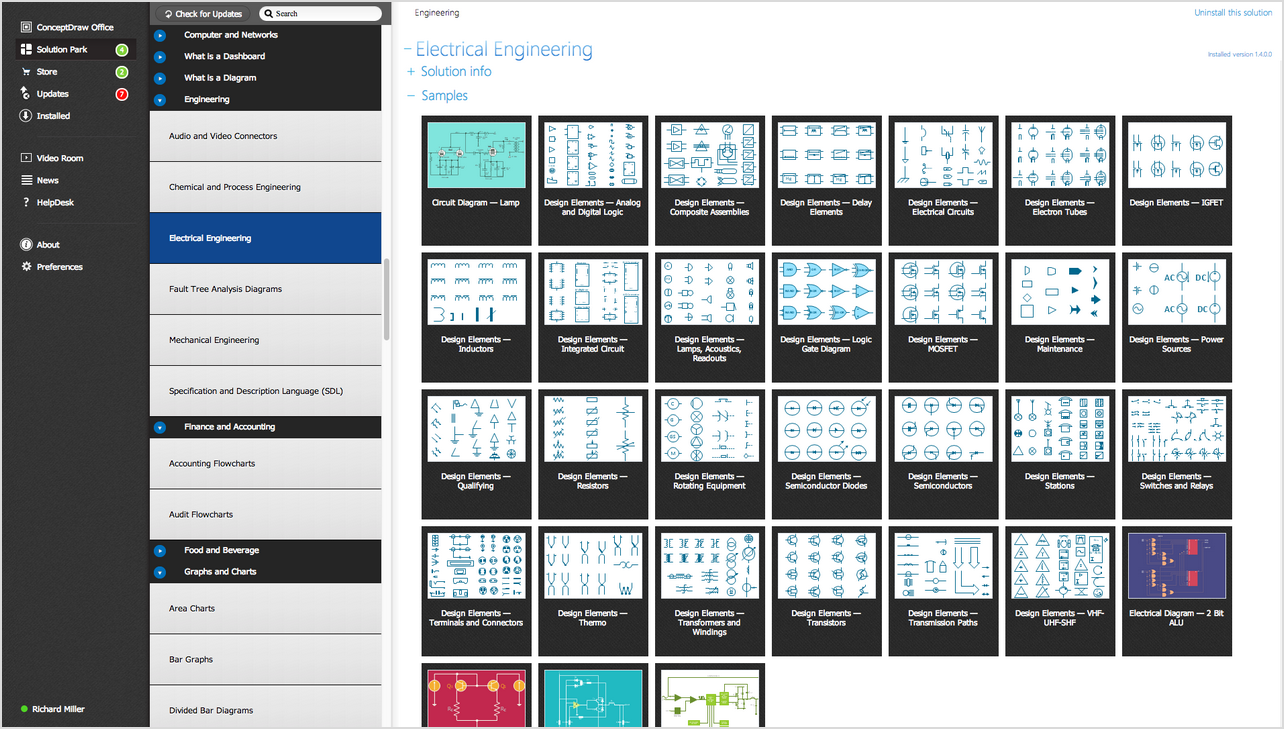ConceptDraw
DIAGRAM 17

# Electrical Symbols, Electrical Diagram Symbols

Drawing electrical circuit diagrams, you will need to represent various electrical and electronic devices (such as batteries, wires, resistors, and transistors) as pictograms called electrical symbols.

Traditionally these symbols may vary from country to country, but today they are standardized internationally to a large extent.

How to create Electrical Diagram using ConceptDraw DIAGRAM. Depending on type of electrical diagram it require variations of Electrical Symbols set. Most of symbols located in the libraries of Electrical Engineering Solution - electrical diagram symbols from the Industrial Engineering Area at the ConceptDraw Solution Park.Example 1. Electrical Symbols: the libraries of Electrical Diagram Symbols in ConceptDraw STORE

Electrical Engineering Solution of ConceptDraw Solution Park includes the set of electrical engineering samples, which can be used in ConceptDraw DIAGRAM There are 26 libraries of electrical symbols. It includes 926 electrical schematic symbols of variety eectrical diagram applications.